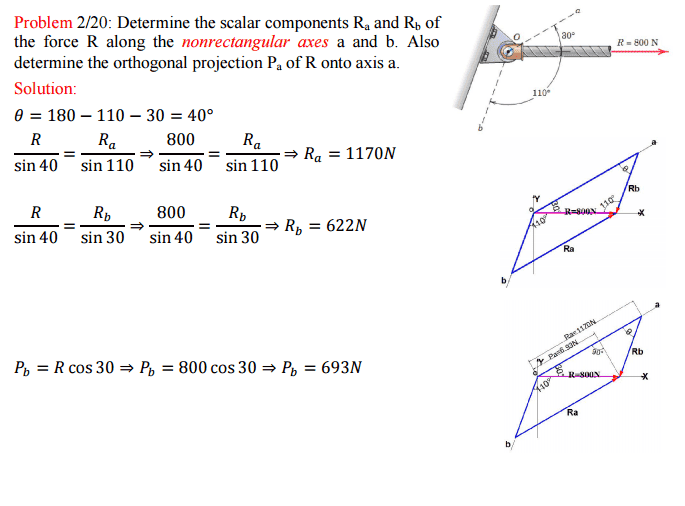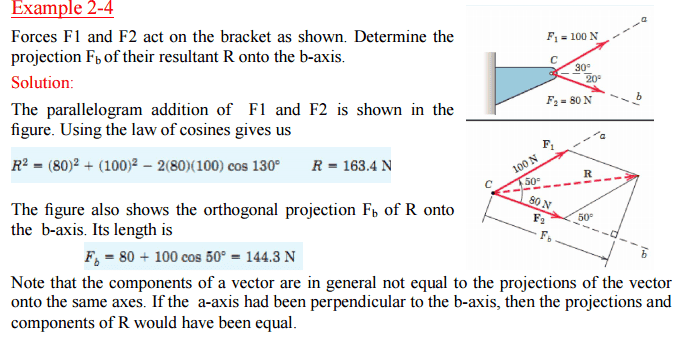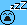# Statics Question: Projection

## Homework Statement

What's the projection of the resultant force onto an said axis?

## Homework Equations

Sine Law, Cosine Law

## The Attempt at a Solution

For problem 2/20, the projection onto the b axis was found by multiplying the resultant force by the cosine of 30 degrees. Why does this method not work for problem 2/4? Why do we need to add in the b-component of the resultant force? The questions are uploaded as pictures along with the solutions. Thank you!## Answers and Replies

BvU
Science Advisor
Homework Helper
Hello fl,Nice pictures. I can imagine you don't want to copy all the typesetting and the pictures. But I can't really imagine what it is that you could want from a possible helper !Ahhh, the problem was in the solution... Goes to show how well posts are being read by helpful helpers who just got out of bed. Fortunately haru has had his lunch already !

Last edited:
haruspex
Science Advisor
Homework Helper
Gold Member
2020 Award
For problem 2/20, the projection onto the b axis was found
No, it is the projection onto the a axis. The references to Pb in the equations are wrong.
Also, in 2/20, R is not shown as being a resultant of other forces; it is the only force.
Why do we need to add in the b-component of the resultant force?
It is not adding a b component of R. The calculation of the projection onto the b axis here does not use R at all. It goes back to the two constituent forces and adds their b components.
This should equal the b component of R. To check that, you would need to find the angle R makes to the b axis. It seems to be a bit less than 30 degrees.

No, it is the projection onto the a axis. The references to Pb in the equations are wrong.
Also, in 2/20, R is not shown as being a resultant of other forces; it is the only force.

It is not adding a b component of R. The calculation of the projection onto the b axis here does not use R at all. It goes back to the two constituent forces and adds their b components.
This should equal the b component of R. To check that, you would need to find the angle R makes to the b axis. It seems to be a bit less than 30 degrees.

Yes sorry! I meant to say the a axis on problem 2/20. Also for 2/4, I was talking about the b-component of the 100N Force. Sorry I made this post before going to bed hence the number of mistakes. Let me clear up my question since it sounds a bit confusing. So for problem 2/20, the projection was found using simple trigonometry, without the additional component while the case wasn't the same for 2/4. For 2/4, we needed to solve for the b-component of the 100N Force, 100*cos(50), and add that to F2 which is in line with the b axis. So what I don't get is why is the approach to each question different? Aren't they basically the same question with just different angles and values? Why can't we find the projection for 2/4 using the same method? Why doesn't 80*cos(20) give us the projection onto the b axis?

haruspex
Science Advisor
Homework Helper
Gold Member
2020 Award
Why doesn't 80*cos(20) give us the projection onto the b axis?
The 80N is F2. That acts along the b axis, so its projection onto the b axis is still 80N.
80cos20 would give its projection onto the horizontal axis.

haruspex
Science Advisor
Homework Helper
Gold Member
2020 Award
Aren't they basically the same question with just different angles and values?
It is the same approach.
In 2/20 you have one known force at a known angle to the desired axis. In 2/4 you have two forces at known angles to the desired axis. In each case you can describe the approach as ΣFicos(θi), where θi is the angle Fi makes to the projection axis.

The 80N is F2. That acts along the b axis, so its projection onto the b axis is still 80N.
80cos20 would give its projection onto the horizontal axis.
Oh sorry, I meant why does Rcos(20) not give us the projection for 2/4?

haruspex
Science Advisor
Homework Helper
Gold Member
2020 Award
Oh sorry, I meant why does Rcos(20) not give us the projection for 2/4?
Because the angle between R and the b axis is not 20°. As I posted, it is a little under 30°.

Oh okay. I see where my fault lies now. It's because I assumed that R is completely horizontal with an angle of 0 degrees in reference to the dotted lines in reference to the first picture in problem 2/4. Thank you so much!

oh and one last question pls. Why does cosine law work for 2/4 when solving for R but when I try to use sine law I get a different value?